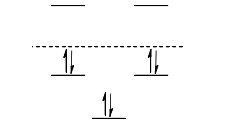# Problem: For the energy diagram shown below:  1) There are 3 antibonding orbitals and 2 bonding orbitals 2) There are 2 antibonding orbitals and 2 non-bonding orbitals and 1 bonding orbital 3) There are 2 antibonding orbitals and  3 bonding orbitals 4) There are 2 antibonding orbitals and 2 non-bonding orbitals and 1 bonding orbital 5) There is 1 bonding orbital and 4 antibonding orbitals

84% (83 ratings)
###### Problem Details

For the energy diagram shown below:

1) There are 3 antibonding orbitals and 2 bonding orbitals

2) There are 2 antibonding orbitals and 2 non-bonding orbitals and 1 bonding orbital

3) There are 2 antibonding orbitals and  3 bonding orbitals

4) There are 2 antibonding orbitals and 2 non-bonding orbitals and 1 bonding orbital

5) There is 1 bonding orbital and 4 antibonding orbitalsFrequently Asked Questions

What scientific concept do you need to know in order to solve this problem?

Our tutors have indicated that to solve this problem you will need to apply the Frost Circle concept. You can view video lessons to learn Frost Circle. Or if you need more Frost Circle practice, you can also practice Frost Circle practice problems.

What is the difficulty of this problem?

Our tutors rated the difficulty ofFor the energy diagram shown below:  1) There are 3 antibon...as high difficulty.

How long does this problem take to solve?

Our expert Organic tutor, Jonathan took 3 minutes and 2 seconds to solve this problem. You can follow their steps in the video explanation above.